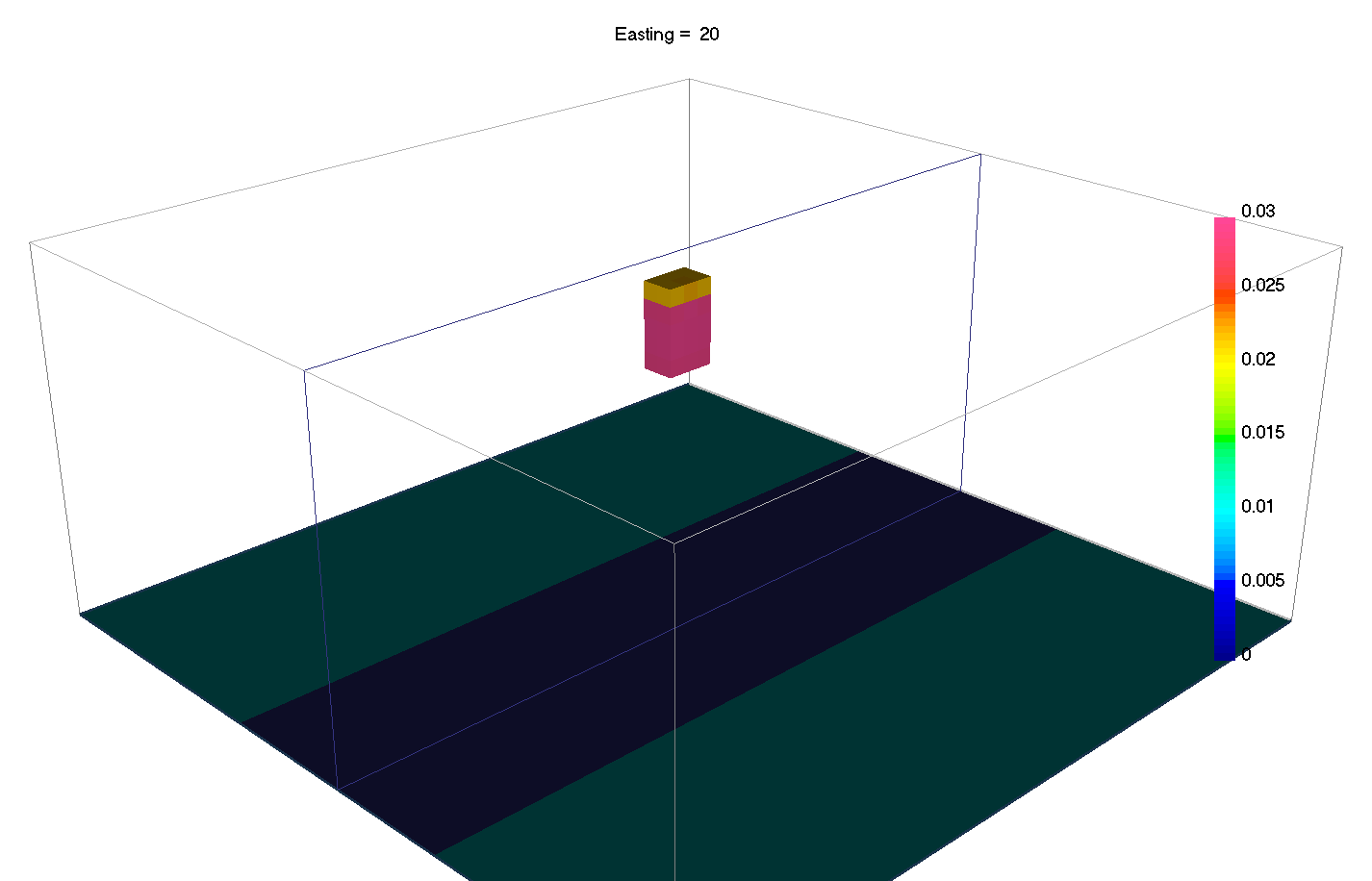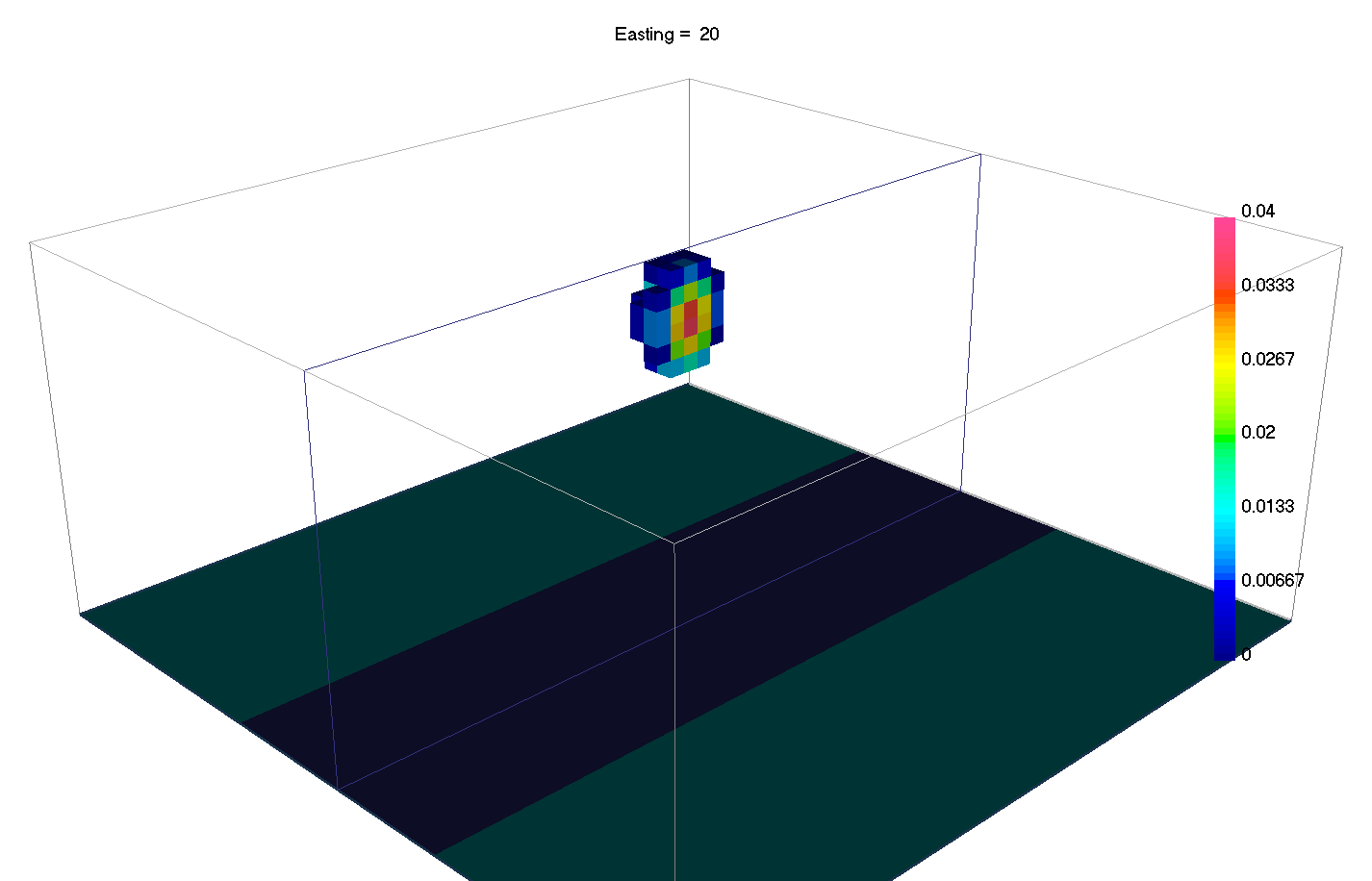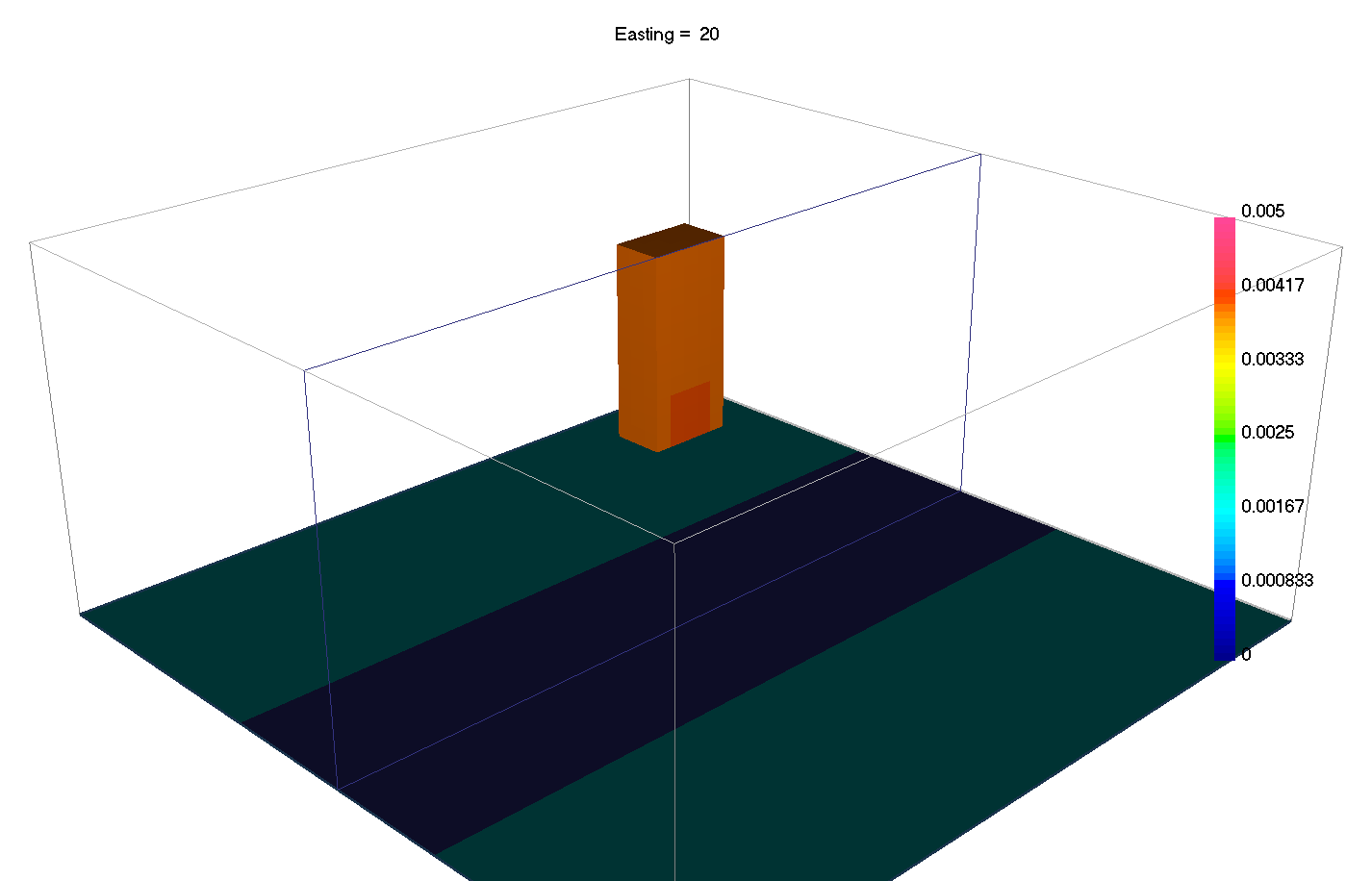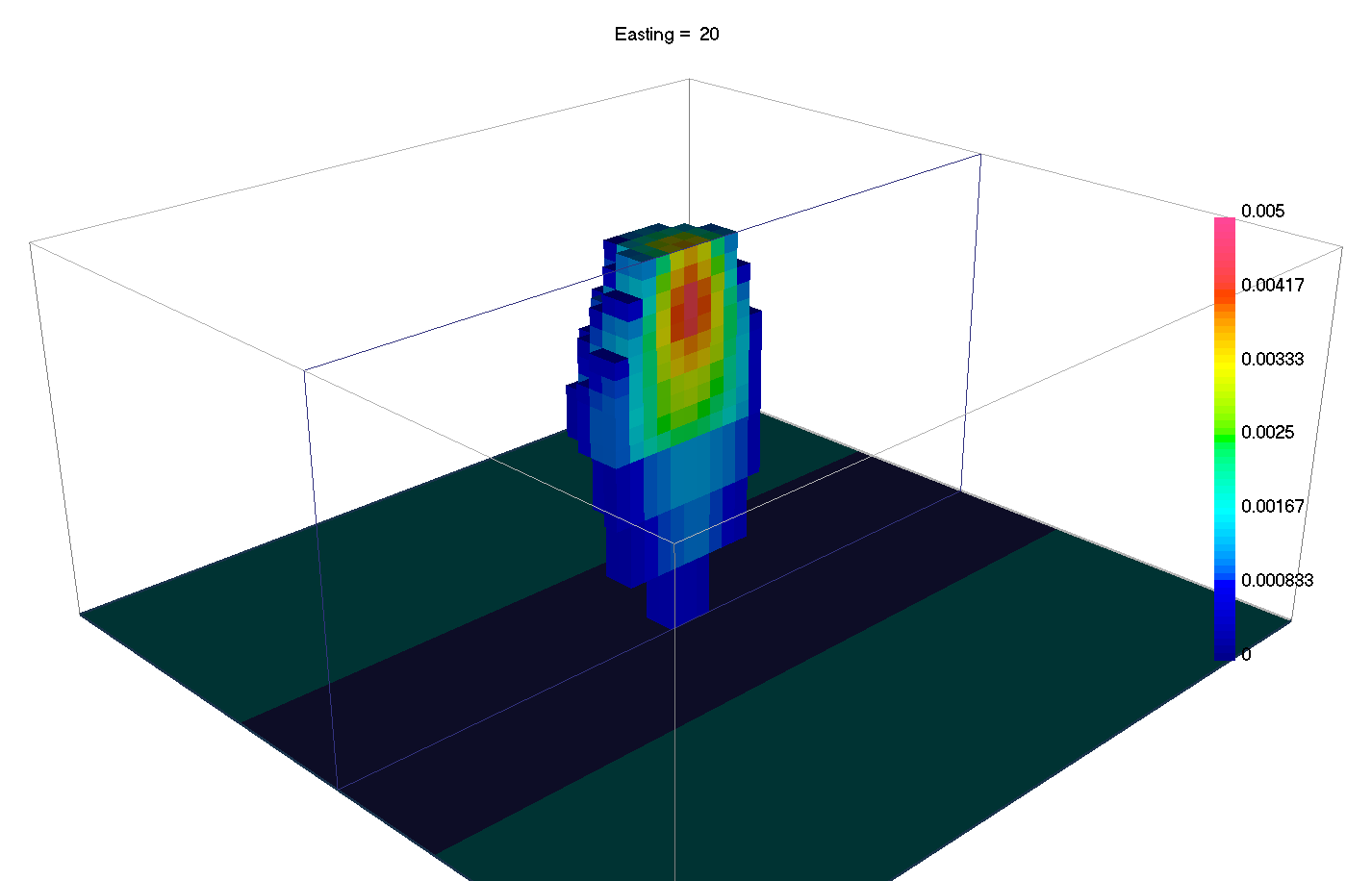# 6.7. Sparse and Blocky Norms¶

Introduced in v6 of the gravity and magnetics codes, it is now possible to change the norms in the different part of the regularization independently. In general terms, a small $$l_p$$-norm applied on the model yields sparse solutions, while small $$l_p$$-norm on the gradients yield blocky solutions.

(1)$\begin{split}\phi_m(\mathbf{m}) = &{\alpha_s} ||\mathbf{W_s}\;\mathbf{\color{blue}R}_s(\mathbf{m}-\mathbf{m}_{ref})||_2^2 +\\ &{\alpha_x} ||\mathbf{W_x}\;\mathbf{\color{blue}R}_x \; \mathbf{G}_x(\mathbf{m}-\mathbf{m}_{ref})||_2^2 +\\ &{\alpha_y} ||\mathbf{W_y}\;\mathbf{\color{blue}R}_y \; \mathbf{G}_y(\mathbf{m}-\mathbf{m}_{ref})||_2^2 +\\ &{\alpha_z} ||\mathbf{W_z}\;\mathbf{\color{blue}R}_z \; \mathbf{G}_z(\mathbf{m}-\mathbf{m}_{ref})||_2^2\end{split}$
 True model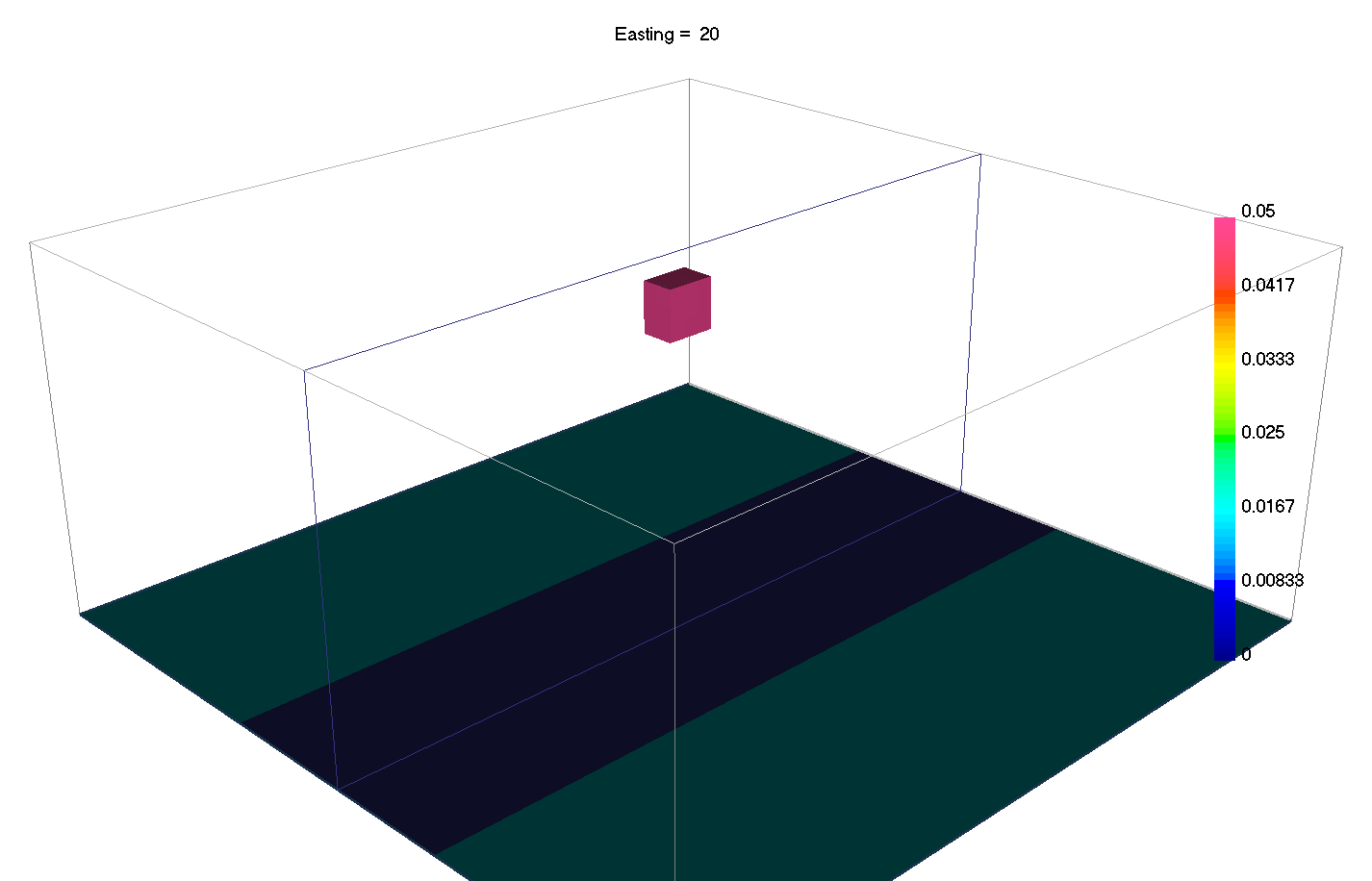Smooth [ps=px=py=pz=2] Sparse [ps=0, px=py=pz=2] Blocky [ps=2, px=py=pz=0] Sparse+blocky [ps=0, px=py=pz=1]

Most codes thus far have involved the $$l_2$$-norm which favors values that are small. Different norms tend to favor models with fewer non-zero model parameters, potentially resulting in simpler, more compact solutions. In discrete form, the general $$l_p$$-norm is written as:

$\begin{split}\| \mathbf{x}(\mathbf{m}) \|^p_p =& \sum_{i=1}^{nC} {|x_i|}^p \\\end{split}$

which we approximate with a Scaled-IRLS methods as

$\sum_{i=1}^{nC} r_i \; x_i^2$

where

${r}_i = {\epsilon_p}^{(1-p/2)}{\Big[ {({x_i}^{(k-1)})}^{2} + \epsilon_p^2 \Big]}^{(p/2 - 1)}$

such $$k$$ denotes the iteration number and $$p$$ and $$\epsilon$$ are sparsity and threshold parameters respectively. A total of 6 variables can be manually adjusted by the user ($$p_s\;, p_x\;, p_y\;, p_z\;, \epsilon_s, \epsilon_{xyz}$$). The choice of $$p$$ and $$\epsilon$$ values is made based upon prior knowledge.